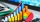Freedom

In the city are 3/9 of women married for 3/6 men. What proportion of the townspeople is free (not married)?

Express as a decimal number.

Result

x =  0.66

Solution:Leave us a comment of this math problem and its solution (i.e. if it is still somewhat unclear...):Be the first to comment!To solve this verbal math problem are needed these knowledge from mathematics:

Do you have a linear equation or system of equations and looking for its solution? Or do you have quadratic equation?

Next similar math problems:

1. MushroomsEva and Jane collected 114 mushrooms together. Eve found twice as much as Jane. How many mushrooms found each of them?
2. Football seasonDalibor and Adam together scored 97 goals in the season. Adam scored 9 goals more than Dalibor. How many goals scored each?
3. Substitutionsolve equations by substitution: x+y= 11 y=5x-25
4. MedianThe number of missed hours was recorded in 11 pupils: 5,12,6,8,10,7,5,110,2,5,6. Determine the median.
5. Reciprocal equationDetermine the root of the equation: 9/x-7/x=1
6. Elimination methodSolve system of linear equations by elimination method: 5/2x + 3/5y= 4/15 1/2x + 2/5y= 2/15
7. Three figures - numbersThe sum of three numbers, if each is 10% larger than the previous one, is 662. Determine the figures.
8. Vijuviju has 40 chickens and rabbits. If in all there are 90 legs. How many rabbits are there with viju??
9. Three brothersThe three brothers have a total of 42 years. Jan is five years younger than Peter and Peter is 2 years younger than Michael. How many years has each of them?
10. Dining roomThe dining room has 11 tables (six and eight seats). In total there are 78 seats in the dining room. How many are six-and eight-seat tables?
11. ClassroomThere are eighty more girls in the class than boys. Boys are 40 percent and girls are 60 percent. How many are boys and how many girls?
12. EquationsSolve following system of equations: 6(x+7)+4(y-5)=12 2(x+y)-3(-2x+4y)=-44
13. TreesAlong the road were planted 250 trees of two types. Cherry for 60 CZK apiece and apple 50 CZK apiece. The entire plantation cost 12,800 CZK. How many was cherries and apples?
14. PearsAndrew, Lenka and Rasto have together 232 pears. Lenka has 28 more than Rasto and Rasto pears have 96 more than Andrew. Determine how much each of them has pears.
15. Liters od milkThe cylinder-shaped container contains 80 liters of milk. Milk level is 45 cm. How much milk will in the container, if level raise to height 72 cm?
16. Three unknownsSolve the system of linear equations with three unknowns: A + B + C = 14 B - A - C = 4 2A - B + C = 0
17. Football match 4In a football match with the Italy lost 3 goals with Germans. Totally fell 5 goals in the match. Determine the number of goals of Italy and Germany.